import pandas as pd
import matplotlib.pyplot as plt
import seaborn as sns

plt.rcParams['figure.figsize'] = (10, 5)


# Count plots and bar plots

### Count plots

In this exercise, we'll return to exploring our dataset that contains the responses to a survey sent out to young people. We might suspect that young people spend a lot of time on the internet, but how much do they report using the internet each day? Let's use a count plot to break down the number of survey responses in each category and then explore whether it changes based on age.

survey_data = pd.read_csv('./dataset/young-people-survey-responses.csv', index_col=0)

Music Techno Movies History Mathematics Pets Spiders Loneliness Parents' advice Internet usage Finances Age Siblings Gender Village - town Age Category Interested in Math
0 5.0 1.0 5.0 1.0 3.0 4.0 1.0 3.0 4.0 few hours a day 3.0 20.0 1.0 female village Less than 21 False
1 4.0 1.0 5.0 1.0 5.0 5.0 1.0 2.0 2.0 few hours a day 3.0 19.0 2.0 female city Less than 21 True
2 5.0 1.0 5.0 1.0 5.0 5.0 1.0 5.0 3.0 few hours a day 2.0 20.0 2.0 female city Less than 21 True
3 5.0 2.0 5.0 4.0 4.0 1.0 5.0 5.0 2.0 most of the day 2.0 22.0 1.0 female city 21+ True
4 5.0 2.0 5.0 3.0 2.0 1.0 1.0 3.0 3.0 few hours a day 4.0 20.0 1.0 female village Less than 21 False
sns.catplot(x='Internet usage', data=survey_data, kind='count', aspect=2);
plt.tight_layout();sns.catplot(y="Internet usage", data=survey_data, kind="count");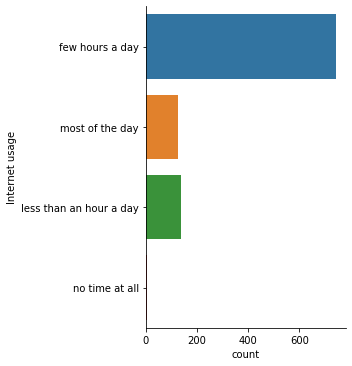sns.catplot(y="Internet usage", data=survey_data, kind="count", col='Age Category');### Bar plots with percentages

Let's continue exploring the responses to a survey sent out to young people. The variable "Interested in Math" is True if the person reported being interested or very interested in mathematics, and False otherwise. What percentage of young people report being interested in math, and does this vary based on gender? Let's use a bar plot to find out.

sns.catplot(x='Gender', y='Interested in Math', data=survey_data, kind='bar');When the y-variable is True/False, bar plots will show the percentage of responses reporting True. This plot shows us that males report a much higher interest in math compared to females.

### Customizing bar plots

In this exercise, we'll explore data from students in secondary school. The "study_time" variable records each student's reported weekly study time as one of the following categories: "<2 hours", "2 to 5 hours", "5 to 10 hours", or ">10 hours". Do students who report higher amounts of studying tend to get better final grades? Let's compare the average final grade among students in each category using a bar plot.

student_data = pd.read_csv('./dataset/student-alcohol-consumption.csv', index_col=0)

school sex age famsize Pstatus Medu Fedu traveltime failures schoolsup ... goout Dalc Walc health absences G1 G2 G3 location study_time
0 GP F 18 GT3 A 4 4 2 0 yes ... 4 1 1 3 6 5 6 6 Urban 2 to 5 hours
1 GP F 17 GT3 T 1 1 1 0 no ... 3 1 1 3 4 5 5 6 Urban 2 to 5 hours
2 GP F 15 LE3 T 1 1 1 3 yes ... 2 2 3 3 10 7 8 10 Urban 2 to 5 hours
3 GP F 15 GT3 T 4 2 1 0 no ... 2 1 1 5 2 15 14 15 Urban 5 to 10 hours
4 GP F 16 GT3 T 3 3 1 0 no ... 2 1 2 5 4 6 10 10 Urban 2 to 5 hours

5 rows × 29 columns

sns.catplot(x='study_time', y='G3', data=student_data, kind='bar');sns.catplot(x="study_time", y="G3", data=student_data, kind="bar",
order = [
'<2 hours',
'2 to 5 hours',
'5 to 10 hours',
'>10 hours'
]);sns.catplot(x="study_time", y="G3",
data=student_data,
kind="bar",
order=["<2 hours",
"2 to 5 hours",
"5 to 10 hours",
">10 hours"],
ci=None);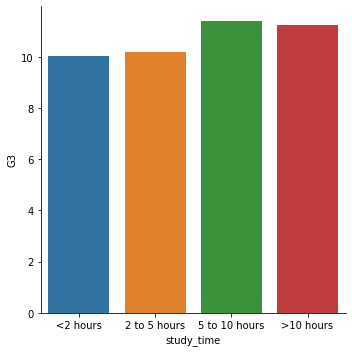Students in our sample who studied more have a slightly higher average grade, but it's not a strong relationship.

## Box plots

• What is a box plot?
• Shows the distribution of quantitative data
• See median, spread, skewness, and outliers
• Facilitates comparisons between groups

### Create and interpret a box plot

Let's continue using the student_data dataset. In an earlier exercise, we explored the relationship between studying and final grade by using a bar plot to compare the average final grade ("G3") among students in different categories of "study_time".

study_time_order = ["<2 hours", "2 to 5 hours",
"5 to 10 hours", ">10 hours"]

# Create a box plot and set the order of the categories
sns.catplot(x='study_time', y='G3',
data=student_data,
kind='box',
order=study_time_order);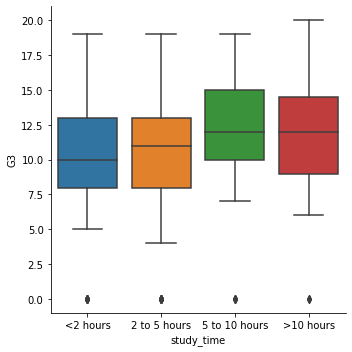### Omitting outliers

Now let's use the student_data dataset to compare the distribution of final grades ("G3") between students who have internet access at home and those who don't. To do this, we'll use the "internet" variable, which is a binary (yes/no) indicator of whether the student has internet access at home.

Since internet may be less accessible in rural areas, we'll add subgroups based on where the student lives. For this, we can use the "location" variable, which is an indicator of whether a student lives in an urban ("Urban") or rural ("Rural") location.

sns.catplot(x='internet', y='G3',
data=student_data,
kind='box',
hue='location',
sym='');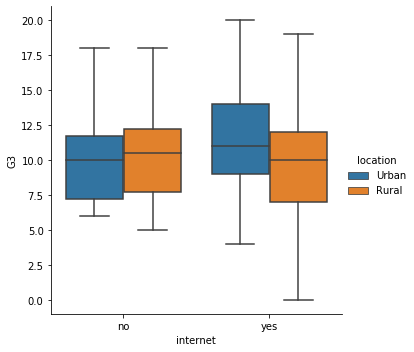The median grades are quite similar between each group, but the spread of the distribution looks larger among students who have internet access.

In the lesson we saw that there are multiple ways to define the whiskers in a box plot. In this set of exercises, we'll continue to use the student_data dataset to compare the distribution of final grades ("G3") between students who are in a romantic relationship and those that are not. We'll use the "romantic" variable, which is a yes/no indicator of whether the student is in a romantic relationship.

Let's create a box plot to look at this relationship and try different ways to define the whiskers.

sns.catplot(x="romantic", y="G3",
data=student_data,
kind="box",
whis=0.5);sns.catplot(x="romantic", y="G3",
data=student_data,
kind="box",
whis=[5, 95]);sns.catplot(x="romantic", y="G3",
data=student_data,
kind="box",
whis=[0, 100]);The median grade is the same between these two groups, but the max grade is higher among students who are not in a romantic relationship.

## Point plots

• What are point plots?
• Points show mean of quantitative variable
• Vertical lines show 95% condence intervals
• Point plots vs. line plots
• Both show:
• Mean of quantitative variable
• 95% condence intervals for the mean
• Differences:
• Line plot has quantitative variable (usually time) on x-axis
• Point plot has categorical variable on x-axis
• Point plots vs. bar plots
• Both show:
• Mean of quantitative variable
• 95% condence intervals for the mean

### Customizing point plots

Let's continue to look at data from students in secondary school, this time using a point plot to answer the question: does the quality of the student's family relationship influence the number of absences the student has in school? Here, we'll use the "famrel" variable, which describes the quality of a student's family relationship from 1 (very bad) to 5 (very good).

sns.catplot(x='famrel', y='absences',
data=student_data,
kind='point');sns.catplot(x="famrel", y="absences",
data=student_data,
kind="point",
capsize=0.2);sns.catplot(x="famrel", y="absences",
data=student_data,
kind="point",
capsize=0.2,
join=False);While the average number of absences is slightly smaller among students with higher-quality family relationships, the large confidence intervals tell us that we can't be sure there is an actual association here.

### Point plots with subgroups

Let's continue exploring the dataset of students in secondary school. This time, we'll ask the question: is being in a romantic relationship associated with higher or lower school attendance? And does this association differ by which school the students attend? Let's find out using a point plot.

sns.catplot(x='romantic', y='absences',
data=student_data,
kind='point',
hue='school');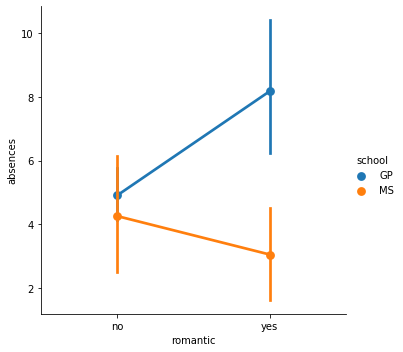sns.catplot(x="romantic", y="absences",
data=student_data,
kind="point",
hue="school",
ci=None);import numpy as np
# Plot the median number of absences instead of the mean
sns.catplot(x="romantic", y="absences",
data=student_data,
kind="point",
hue="school",
ci=None,
estimator=np.median);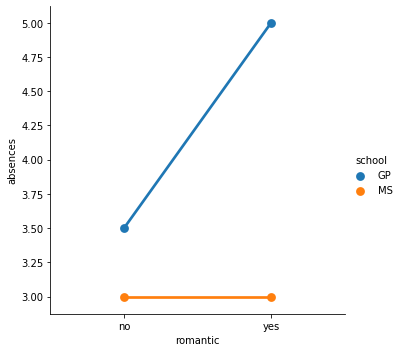It looks like students in romantic relationships have a higher average and median number of absences in the GP school, but this association does not hold for the MS school.